Question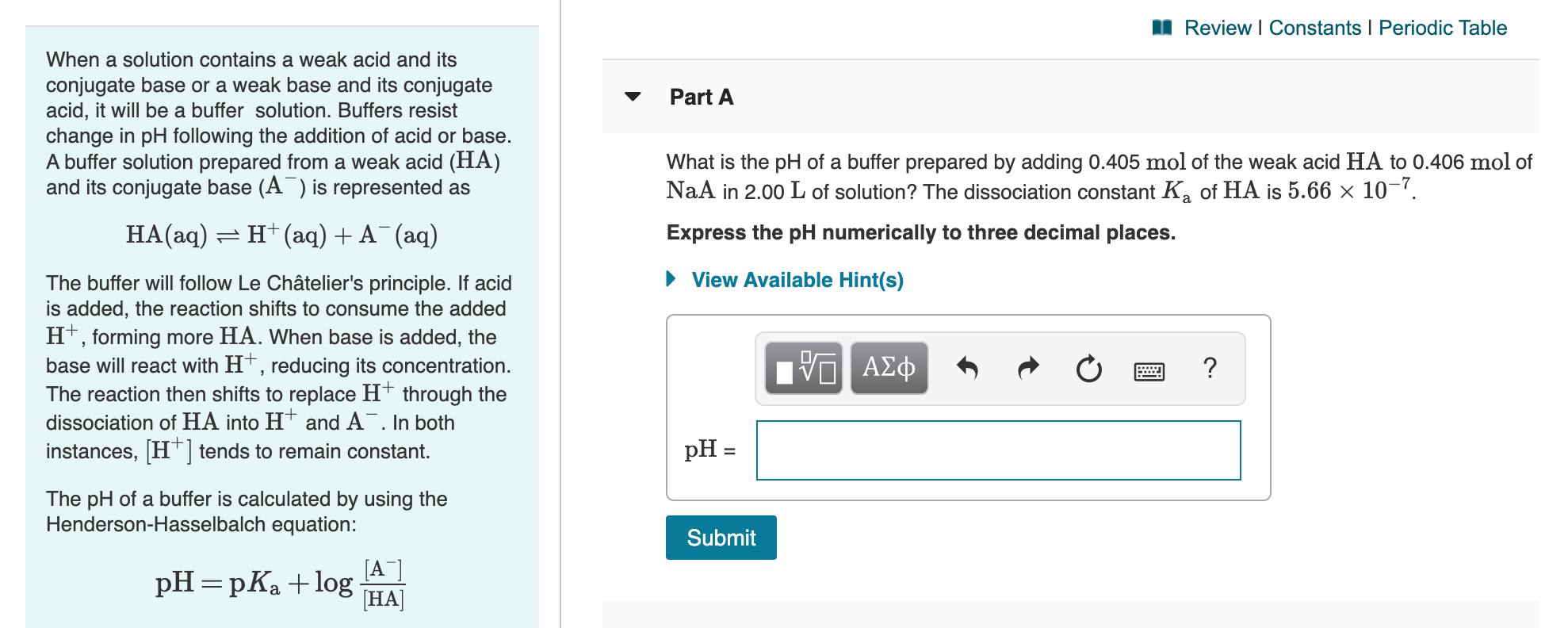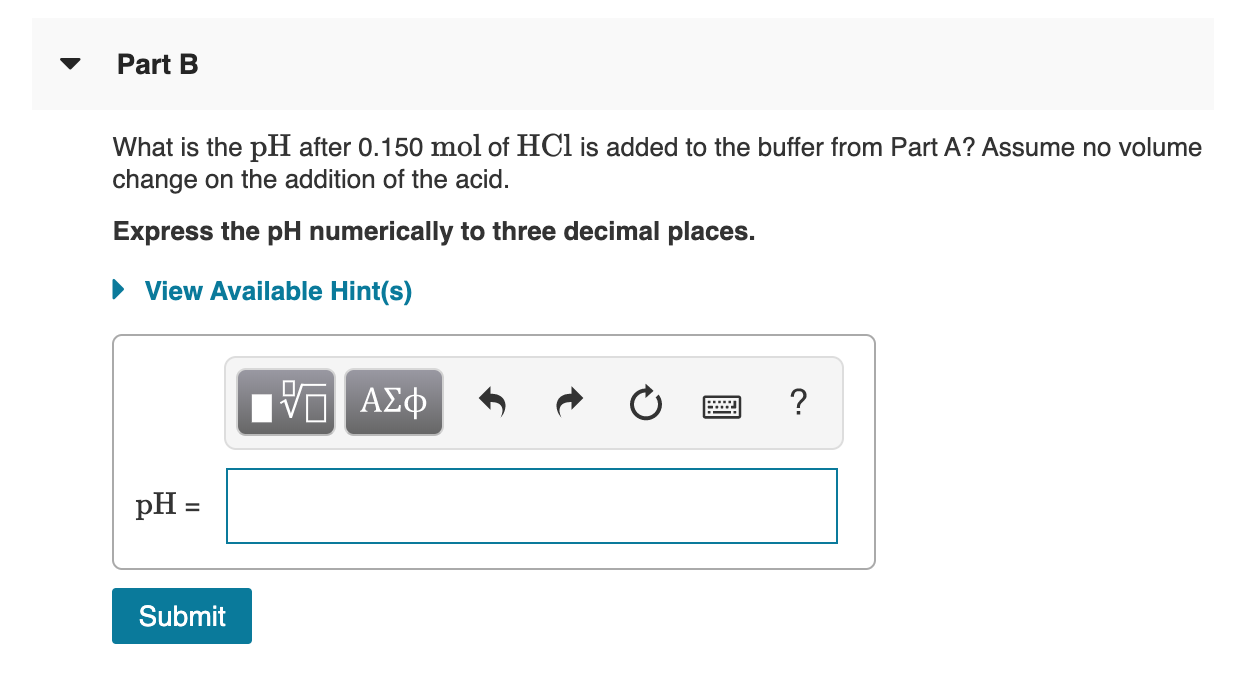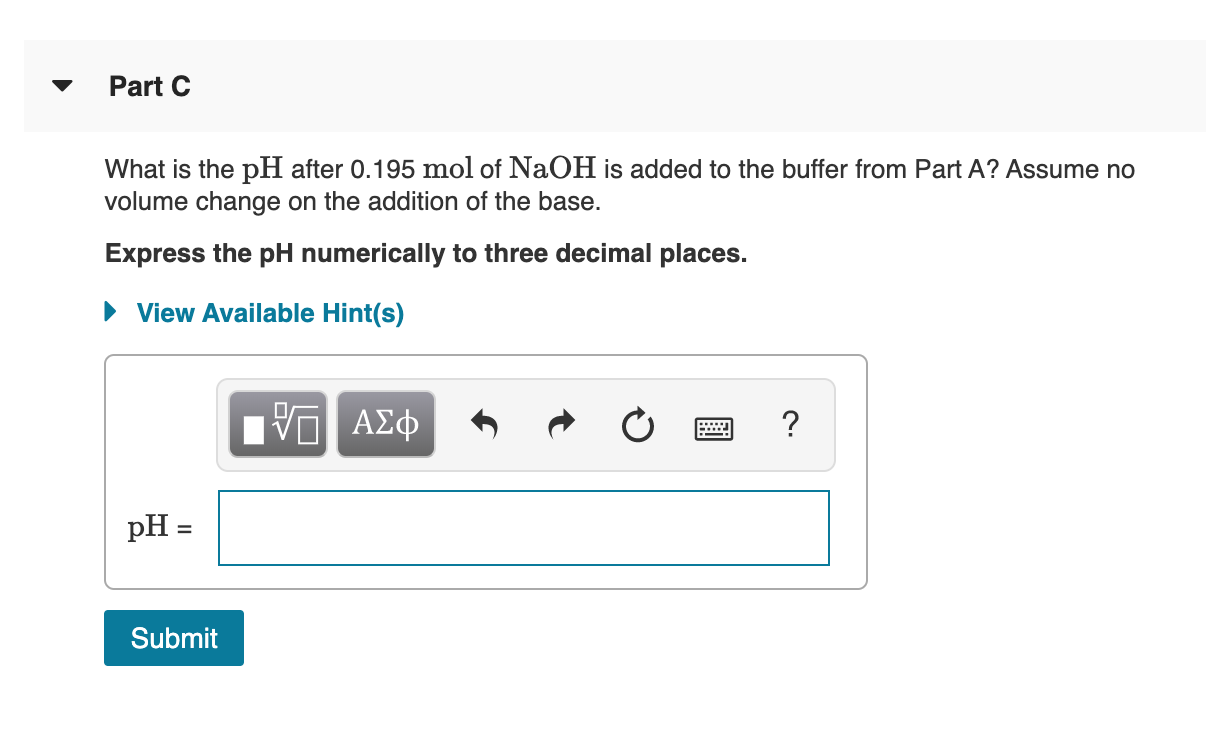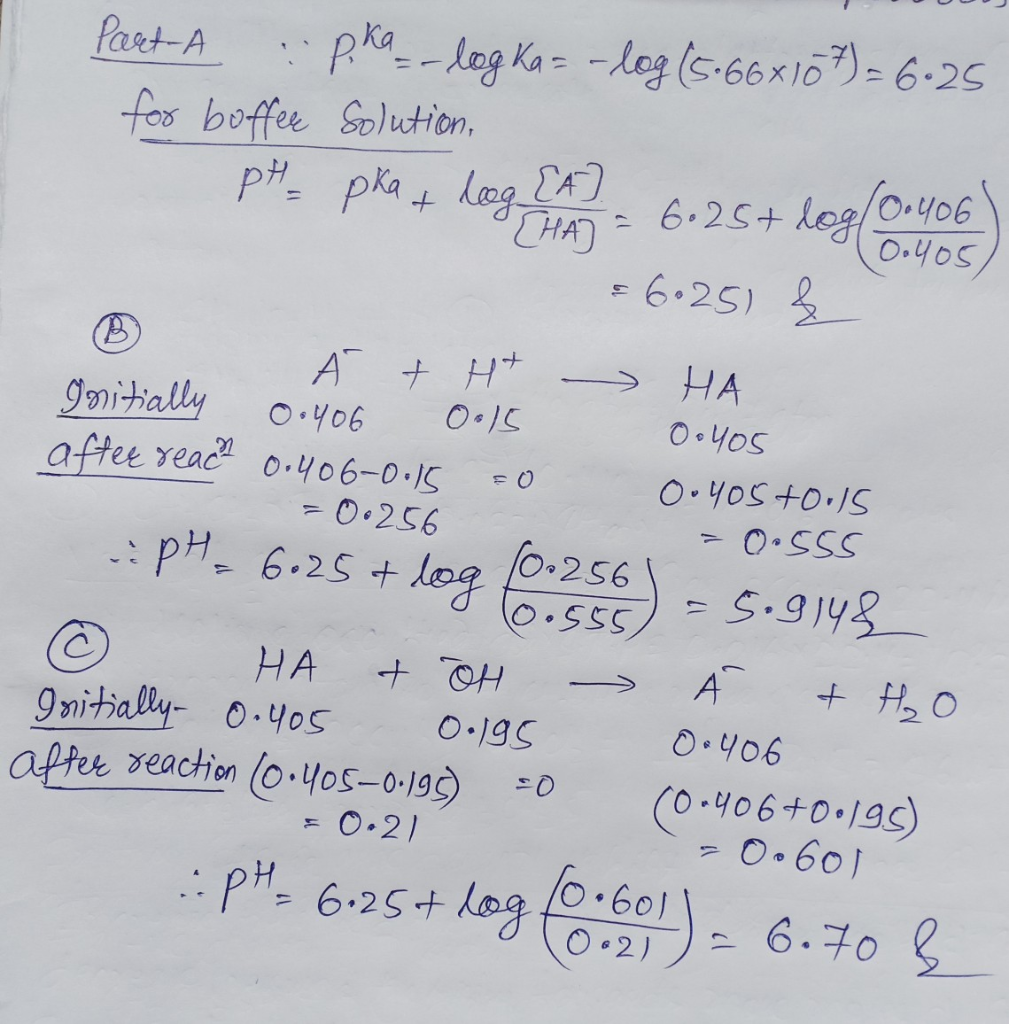#### Earn Coins

Coins can be redeemed for fabulous gifts.

Similar Homework Help Questions
• ### ( 12 0127 Review Constants Periodic T What is the ph of a butler prepared by...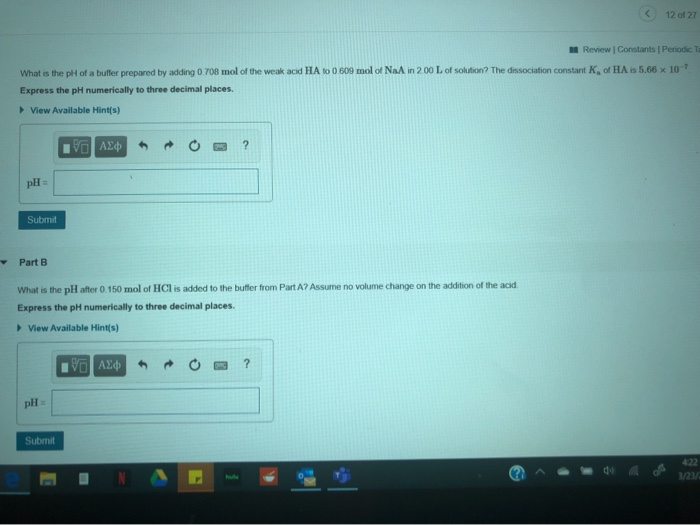( 12 0127 Review Constants Periodic T What is the ph of a butler prepared by adding you mol of the weak acid HA 10 0 609 mol of NaA in 200 L of solution? The dissociation constant of HAI 5.66 x 10 Express the pH numerically to three decimal places View Available Hints) AED + O ? Submit Part B What is the pH after 0. 150 mol of HCl is added to the butter from PartA? Assume no...

• ### Part A - 7 What is the pH of a buffer prepared by adding 0.809 mol...Part A - 7 What is the pH of a buffer prepared by adding 0.809 mol of the weak acid HA to 0.507 mol of NaA in 2.00 L of solution? The dissociation constant K, of HA is 5.66 x 10 Express the pH numerically to three decimal places. View Available Hint(s) Ivo AED ? pH = Submit Part B What is the pH after 0.150 mol of HCl is added to the buffer from Part A? Assume no volume...

• ### ± pH Changes in Buffers Part A What is the pH of a buffer prepared by...

± pH Changes in Buffers Part A What is the pH of a buffer prepared by adding 0.708 mol of the weak acid HA to 0.608 mol of NaA in 2.00 L of solution? The dissociation constant Ka of HA is 5.66×10−7. Express the pH numerically to three decimal places. Part B What is the pH after 0.150 mol of HCl is added to the buffer from Part A? Assume no volume change on the addition of the acid. Express...

• ### What is the pH after 0.150 mol of HCl is added to the buffer from Part...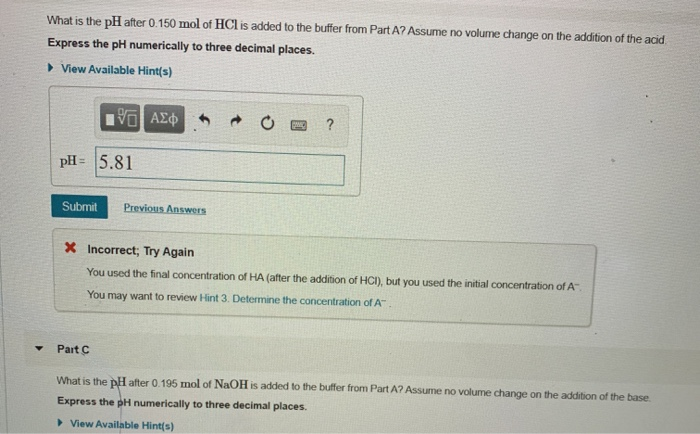What is the pH after 0.150 mol of HCl is added to the buffer from Part A? Assume no volume change on the addition of the acid Express the pH numerically to three decimal places. ► View Available Hint(s) ROO? V AE pH= 5.81 Submit Previous Answers X Incorrect; Try Again You used the final concentration of HA (after the addition of HCI), but you used the initial concentration of A You may want to review Hint 3. Determine the...

• ### Part A What is the pH of a buffer prepared by adding 0.708 mol of the weak acid HA to 0.406 mol of NaA in 2.00 Lof sol...

Part A What is the pH of a buffer prepared by adding 0.708 mol of the weak acid HA to 0.406 mol of NaA in 2.00 Lof solution? The dissociation constant Ka of HA is 5.66×10−7. Express the pH numerically to three decimal places. Part B What is the pH after 0.150 mol of HCl is added to the buffer from Part A? Assume no volume change on the addition of the acid. Express the pH numerically to three decimal...

• ### can someone help with steps plz Sing a Strong Acid to a Butter Review Constants Periodic...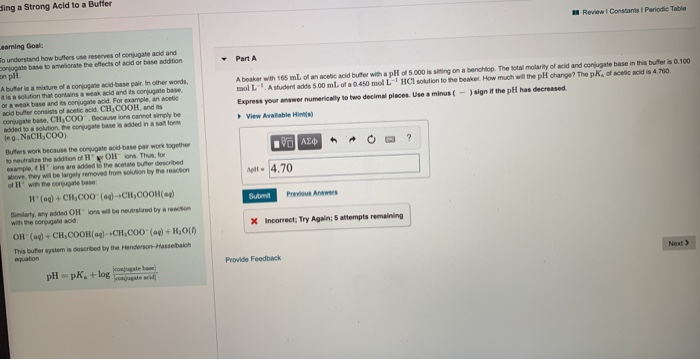can someone help with steps plz Sing a Strong Acid to a Butter Review Constants Periodic Table Part A Abesker with 165 mL of an cetic acid buffer with a pH of 5.000 is siting on a benchtop. The total molarity of acid and conjugate base in this buffer is 0.100 mol L-A student adds 500 ml of a 0.450 mol HCl solution to the beaker. How much will the pH change? The pk, of acetic acid is 4.700 Express...

• ### 5 of 5 > Review I Constants 1 Periodic Table Part A A beaker with 145...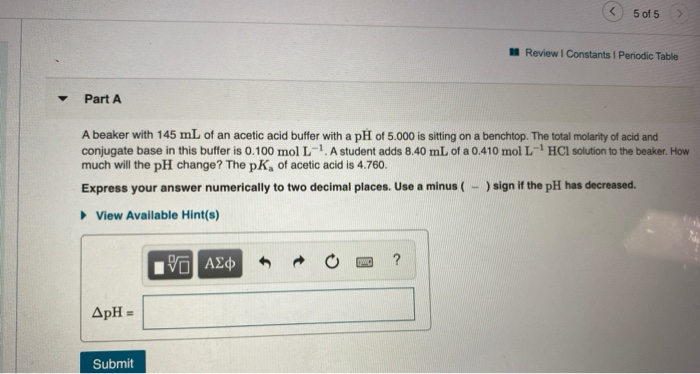5 of 5 > Review I Constants 1 Periodic Table Part A A beaker with 145 mL of an acetic acid buffer with a pH of 5.000 is sitting on a benchtop. The total molarity of acid and conjugate base in this buffer is 0.100 mol L-1. A student adds 8.40 mL of a 0.410 mol L-HCl solution to the beaker. How much will the pH change? The pK, of acetic acid is 4.760. Express your answer numerically to two...

• ### Part A What is the pH of a buffer prepared by adding 0.506 mol of the weak acid HA to 0.608 mol of NaA in 2.00 L of so...

Part A What is the pH of a buffer prepared by adding 0.506 mol of the weak acid HA to 0.608 mol of NaA in 2.00 L of solution? The dissociation constant Ka of HA is 5.66×10−7. Express the pH numerically to three decimal places. pH = SubmitHintsMy AnswersGive UpReview Part Part B What is the pH after 0.150 mol of HCl is added to the buffer from Part A? Assume no volume change on the addition of the acid....

• ### Constants 1 Per A certain weak acid, HA, with a K value of 5.61 x 10-6,...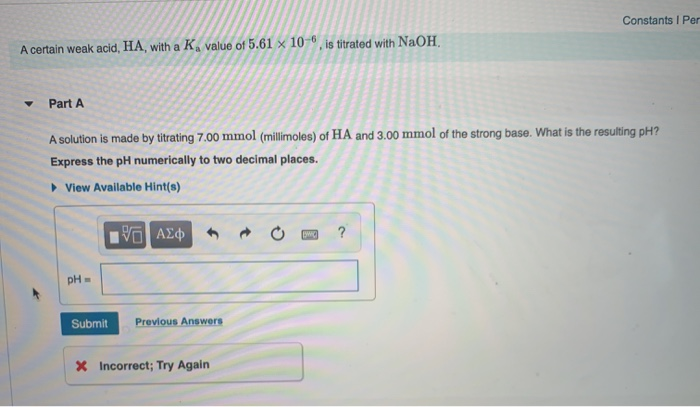Constants 1 Per A certain weak acid, HA, with a K value of 5.61 x 10-6, is titrated with NaOH. Part A A solution is made by titrating 7.00 mmol (millimoles) of HA and 3.00 mmol of the strong base. What is the resulting pH? Express the pH numerically to two decimal places. View Available Hint(s) IVO AQ R O ? pH в Submit Previous Answers X Incorrect; Try Again Part B More strong base is added until the equivalence...

• ### Percent lonization of a Weak Acid 7 of 13 Constants I Periodic Table Part A Pyridine...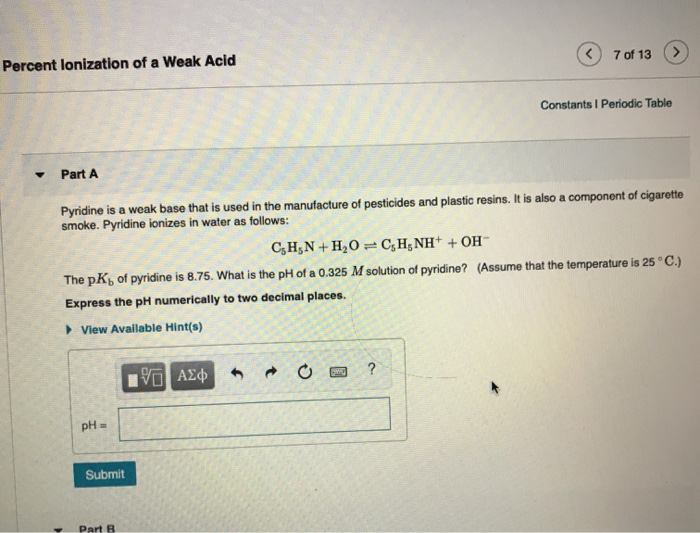Percent lonization of a Weak Acid 7 of 13 Constants I Periodic Table Part A Pyridine is a weak base that is used in the manufacture of pesticides and plastic resins. It is also a component of cigarette smoke. Pyridine ionizes in water as follows: CsHN+H20C,HNH+OH (Assume that the temperature is 25° C.) The pK of pyridine is 8.75. What is the pH of a 0.325 M solution of pyridine? Express the pH numerically to two decimal places. View Available...